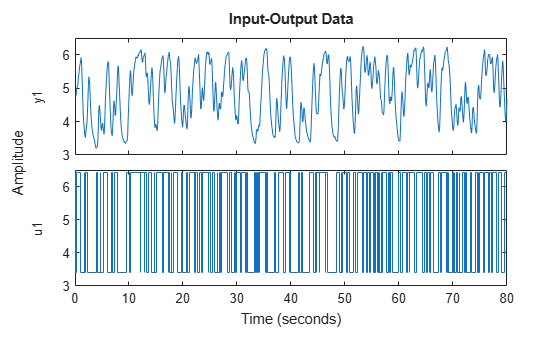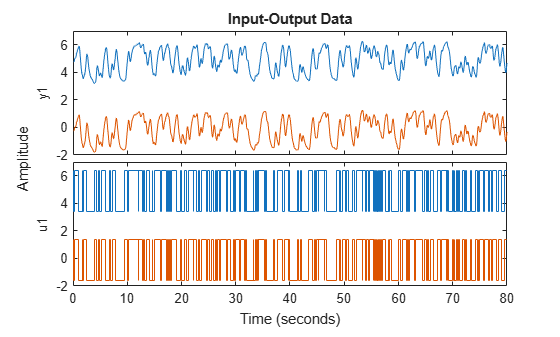# TrendInfo

Offset and linear trend slope values for detrending data

## Description

`TrendInfo` class represents offset and linear trend information of input and output data. Constructing the corresponding object lets you:

• Compute and store mean values or best-fit linear trends of input and output data signals.

• Define specific offsets and trends to be removed from input-output data.

By storing offset and trend information, you can apply it to multiple data sets.

After estimating a linear model from detrended data, you can simulate the model at original operation conditions by adding the saved trend to the simulated output using `retrend`.

## Construction

For transient data, if you want to define a specific offset or trend to be removed from this data, create the `TrendInfo` object using `getTrend`. For example:

`T = getTrend(data)`

where data is the `iddata` object from which you will be removing the offset or linear trend, and `T` is the `TrendInfo` object. You must then assign specific offset and slope values as properties of this object before passing the object as an argument to `detrend`.

For steady-state data, if you want to detrend the data and store the trend information, use the `detrend` command with the output argument for storing trend information.

## Properties

After creating the object, you can use `get` or dot notation to access the object property values.

Property NameDefaultDescription
`DataName``''`Name of the `iddata` object from which trend information is derived (if any)
`InputOffset``zeros(1,nu)`, where `nu` is the number of inputs
• For transient data, the physical equilibrium offset you specify for each input signal.

• For steady-state data, the mean of input values. Computed automatically when detrending the data.

• If removing a linear trend from the input-output data, the value of the line at `t0`, where `t0` is the start time.

For multiple experiment data, this is a cell array of size equal to the number of experiments in the data set.

`InputSlope``zeros(1,nu)`, where `nu` is the number of inputs

Slope of linear trend in input data, computed automatically when using the `detrend` command to remove the linear trend in the data.

For multiple experiment data, this is a cell array of size equal to the number of experiments in the data set.

`OutputOffset``zeros(1,ny)`, where `ny` is the number of outputs
• For transient data, the physical equilibrium offset you specify for each output signal

• For steady-state data, the mean of output values. Computed automatically when detrending the data.

• If removing a linear trend from the input-output data, the value of the line at `t0`, where `t0` is the start time.

For multiple experiment data, this is a cell array of size equal to the number of experiments in the data set.

`OutputSlope``zeros(1,ny)`, where `ny` is the number of outputs

Slope of linear trend in output data, computed automatically when using the `detrend` command to remove the linear trend in the data.

For multiple experiment data, this is a cell array of size equal to the number of experiments in the data set.

## Examples

collapse all

Remove specified offset from input and output signals.

Load SISO data containing vectors `u2` and `y2`.

`load dryer2`

Create a data object with sample time of 0.08 seconds and plot it.

```data = iddata(y2,u2,0.08); plot(data)```The data has a nonzero mean value.

Store the data offset and trend information in a `TrendInfo` object.

`T = getTrend(data);`

Assign offset values to the `TrendInfo` object.

```T.InputOffset = 5; T.OutputOffset = 5;```

Subtract offset from the data.

`data_d = detrend(data,T);`

Plot the detrended data on the same plot.

```hold on plot(data_d)```View the mean value removed from the data.

`get(T)`
```ans = struct with fields: DataName: 'data' InputOffset: 5 OutputOffset: 5 InputSlope: 0 OutputSlope: 0 ```

Construct the `TrendInfo` object that stores trend information as part of data detrending.

Load SISO data containing vectors `u2` and `y2`.

`load dryer2`

Create data object with sample time of 0.08 seconds.

`data = iddata(y2,u2,0.08);`

Detrend the mean from the data and store the mean as a `TrendInfo` object `T`.

`[data_d,T] = detrend(data,0);`

View the mean value removed from the data.

`get(T)`
```ans = struct with fields: DataName: 'data' InputOffset: 5.0000 OutputOffset: 4.8901 InputSlope: 0 OutputSlope: 0 ```